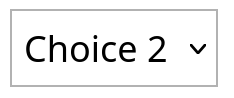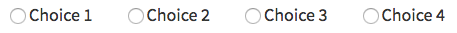# Choose one from a list / Choose several from a list / Match choices with answers¶

There are three kinds of “multiple response” part types in Numbas, with similar settings. They are listed here together.

Multiple response part types

Choose one from a list

The student must choose one of several options

Choose several from a list

The student can choose any of a list of options

The student is presented with a 2D grid of choices and answers. Depending on how the part is set up, they must either match up each choice with an answer, or select any number of choice-answer pairs.

## Marking¶

Minimum marks

If the student would have scored less than this many marks, they are instead awarded this many. Useful in combination with negative marking.

Maximum marks

If the student would have scored more than this many marks, they are instead awarded this many. The value 0 means “no maximum mark”.

For choose several from a list and match choices with answers parts, the student must select at least this many choices. The value 0 means “no minimum”, though the student must make at least one choice to submit the part.

For choose several from a list and match choices with answers parts, the student must select at most this many choices. The value 0 means “no maximum”.

What to do if wrong number of answers selected

If the student selects too few or too many answers, either do nothing, show them a warning but allow them to submit, or prevent submission until they pick an acceptable number of answers.

Shuffle order of choices?

If this is ticked, the choices are displayed in random order.

If this is ticked, the answers are displayed in random order.

Marking method

(Choose several from a list and Match choices with answers, when Selection type is “Checkboxes”, only)

This determines how the student’s score is determined, based on their selections and the marking matrix.

“Sum ticked cells” means that for each checkbox the student ticks, the corresponding entry in the marking matrix is added to their score. Unticked cells are ignored. This marking method is suitable for situations where the student should only select choices they’re sure about. You could apply negative marks for incorrect choices.

“Score per matched cell” means that for each checkbox, the student is awarded an equal proportion of the Maximum marks, if their selection for that cell matches the marking matrix. A positive value in the marking matrix signifies that the student should tick that checkbox, while a value of zero signifies that the student should not tick that box. This marking method is suitable for situations where the student must separate the available choices into two sets.

“All-or-nothing” means that the student is awarded the Maximum marks available if their selection exactly matches the marking matrix, and zero marks otherwise. This marking method is suitable for situations where the student must exactly match a certain pattern, and there is no meaningful “partially correct” answer.

Number of display columns

For choose one from a list and choose several from a list parts, this dictates how many columns the choices are displayed in. If 0, the choices are displayed on a single line, wrapped at the edges of the screen.

Selection type

For match choices with answers parts, “One from each row” means that the student can only select one answer from each row and “Checkboxes” means that the student can select any number of choice-answer pairs.

For choose one from a list parts, users can select only one of the choices.

“Drop down list” means that the choices are shown as a selection box; the student can click to show the choices in a vertical list.Warning

Drop down lists can only display plain text, due to how selection boxes are implemented in HTML. This means that any formatting applied in the editor will not be displayed, and LaTeX will not render properly.

“Radio buttons” means that choices are shown separately, in-line with the part prompt.Custom marking matrix

If the checkbox is ticked, the JME expression in the box below is evaluated and used to assign numbers of marks to choices.

Custom matrix expression

Define the number of marks to award for each of the choices. For choose one from a list and choose several from a list parts, the expression should evaluate to a list of numbers, while for match choices with answers it should evaluate to a list of lists of numbers representing a 2d array, or a matrix object, giving the number of marks to associate with each choice-answer pair.

Layout

Define which choices are available to be picked. If Custom expression is selected, give either a list of lists of boolean values, or a matrix with as many rows as the part has choices and as many columns as the part has answers. Any non-zero value in the matrix indicates that the corresponding choice-answer pair should be available to the student.

Show choice feedback state?

If ticked, choices selected by the student will be highlighted as ‘correct’ if they have a positive score, and ‘incorrect’ if they are worth zero or negative marks. If show score feedback icon? is not ticked, the ticked choices will be given a neutral highlight regardless of their scores.

If this is not ticked, no highlighting will be applied to ticked choices. This is appropriate if the part uses a custom marking algorithm which awards a score based on the set of choices considered as a whole.

## Choices¶

Variable list of choices?

Should the list of choices be defined by a JME expression? If this is ticked, you must give a custom matrix expression.

List of choices

If Variable list of choices? is ticked, this JME expression defines the list of choice strings to display to the student.

Marks (choose one from a list / choose several from a list only)

The number of marks to award (or take away, if you enter a negative number) when the student picks this choice.

Distractor message (choose one from a list / choose several from a list only)

A message to display to the student in the part’s feedback section after they select a particular choice. It can be useful to give some explanation of why a choice is incorrect.

Only Match choices with answers parts have answers as well as choices.

Should the list of answers be defined by a JME expression? If this is ticked, you must give a custom matrix expression.

If Variable list of answers? is ticked, this JME expression defines the list of answer strings to display to the student.

## Marking matrix¶

Only Match choices with answers parts have a marking matrix tab: for the other part types, the marking matrix is defined implicitly by the score for each choice.

Assign marks to each pair of choice and answer using the input boxes.

Custom marking matrix

If the checkbox is ticked, the JME expression in the box below is evaluated and used to assign numbers of marks to choices.

Custom matrix expression

Define the number of marks to award for each of the choices. Either a list of lists representing a 2d array, or a matrix object, giving the number of marks to associate with each choice-answer pair.

## Marking settings¶

This part type provides the following properties to the `settings` object:

maxMarksEnabled

Is there a maximum number of marks the student can get? Set by Maximum marks.

The minimum number of responses the student must select, set by Minimum answers.

The maximum number of responses the student must select, set by Maximum answers.

shuffleChoices

Shuffle order of choices?

matrix

A 2D `list` of marks for each answer/choice pair. Arranged as `settings["matrix"][answer][choice]`.

displayType

Selection type: one of `"radiogroup"`, `"checkbox"` or `"dropdownlist"`.

warningType

What to do if the student picks the wrong number of responses? Either `"none"` (do nothing), `"prevent"` (don’t let the student submit), or `"warn"` (show a warning but let them submit)

layoutType

The type of layout to use, set by Layout. One of `"all"`, `"lowertriangle"`, `"strictlowertriangle"`, `"uppertriangle"`, `"strict uppertriangle"`, `"expression"`.

layoutExpression

`string` form of a JME expression to produce a 2d array or matrix describing the layout when :data`layoutType` is `"expression"`.

## Examples¶

The following questions in the Numbas demo demonstrate a variety of ways of using multiple choice parts.